# Features in detail¶

## Power flow analysis¶

In order to analyse voltages and line loadings a non-linear power flow analysis (PF) is conducted. All loads and generators are modelled as PQ nodes; the slack is modelled as a PV node with a set voltage of 1,p.u. and positioned at the substation’s secondary side.

## Grid expansion¶

### General methodology¶

The grid expansion methodology is conducted in reinforce_grid().

The order grid expansion measures are conducted is as follows:

• Reinforce lines in MV grid due to voltage issues
• Reinforce distribution substations due to voltage issues
• Reinforce lines in LV grid due to voltage issues

Reinforcement of stations and lines due to overloading issues is performed twice, once in the beginning and again after fixing voltage issues, as the changed power flows after reinforcing the grid may lead to new overloading issues. How voltage and overloading issues are identified and solved is shown in figure Grid expansion measures and further explained in the following sections.

reinforce_grid() offers a few additional options. It is e.g. possible to conduct grid reinforcement measures on a copy of the graph so that the original grid topology is not changed. It is also possible to only identify necessary reinforcement measures for two worst-case snapshots in order to save computing time and to set combined or separate allowed voltage deviation limits for MV and LV. See documentation of reinforce_grid() for more information.

Identification of overloading and voltage issues is conducted in check_tech_constraints.

Voltage issues are determined based on allowed voltage deviations set in the config file config_grid_expansion in section grid_expansion_allowed_voltage_deviations. It is possible to set one allowed voltage deviation that is used for MV and LV or define separate allowed voltage deviations. Which allowed voltage deviation is used is defined through the parameter combined_analysis of reinforce_grid(). By default combined_analysis is set to false, resulting in separate voltage limits for MV and LV, as a combined limit may currently lead to problems if voltage deviation in MV grid is already close to the allowed limit, in which case the remaining allowed voltage deviation in the LV grids is close to zero.

Allowed voltage deviations as well as load factors are in most cases different for load and feed-in case. Load and feed-in case are commonly used worst-cases for grid expansion analyses. Load case defines a situation where all loads in the grid have a high demand while feed-in by generators is low or zero. In this case power is flowing from the high-voltage grid to the distribution grid. In the feed-in case there is a high generator feed-in and a small energy demand leading to a reversed power flow. Load and generation assumptions for the two worst-cases are definded in the config file config_timeseries in section worst_case_scale_factor (scale factors describe actual power to nominal power ratio of generators and loads).

When conducting grid reinforcement based on given time series instead of worst-case assumptions, load and feed-in case also need to be definded to determine allowed voltage deviations and load factors. Therefore, the two cases are identified based on the generation and load time series of all loads and generators in the grid and defined as follows:

• Load case: positive (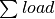-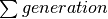)
• Feed-in case: negative (-) -> reverse power flow at HV/MV substation

Grid losses are not taken into account. See assign_load_feedin_case() for more details and implementation.

Exceedance of allowed line load of MV and LV lines is checked in mv_line_load() and lv_line_load(), respectively. The functions use the given load factor and the maximum allowed current given by the manufacturer (see I_max_th in tables LV cables, MV cables and MV overhead lines) to calculate the allowed line load of each LV and MV line. If the line load calculated in the power flow analysis exceeds the allowed line load, the line is reinforced (see Reinforce lines due to overloading issues).

Exceedance of allowed station load of HV/MV and MV/LV stations is checked in hv_mv_station_load() and mv_lv_station_load(), respectively. The functions use the given load factor and the maximum allowed apparent power given by the manufacturer (see S_nom in tables LV transformers, and MV transformers) to calculate the allowed apparent power of the stations. If the apparent power calculated in the power flow analysis exceeds the allowed apparent power the station is reinforced (see Reinforce stations due to overloading issues).

#### Check line and station voltage deviation¶

Compliance with allowed voltage deviation limits in MV and LV grids is checked in mv_voltage_deviation() and lv_voltage_deviation(), respectively. The functions check if the voltage deviation at a node calculated in the power flow analysis exceeds the allowed voltage deviation. If it does, the line is reinforced (see Reinforce MV/LV stations due to voltage issues or Reinforce lines due to voltage).

### Grid expansion measures¶

Reinforcement measures are conducted in reinforce_measures. Whereas overloading issues can usually be solved in one step, except for some cases where the lowered grid impedance through reinforcement measures leads to new issues, voltage issues can only be solved iteratively. This means that after each reinforcement step a power flow analysis is conducted and the voltage rechecked. An upper limit for how many iteration steps should be performed is set in order to avoid endless iteration. By default it is set to 10 but can be changed using the parameter max_while_iterations of reinforce_grid().

Line reinforcement due to overloading is conducted in reinforce_branches_overloading(). In a first step a parallel line of the same line type is installed. If this does not solve the overloading issue as many parallel standard lines as needed are installed.

Reinforcement of HV/MV and MV/LV stations due to overloading is conducted in extend_substation_overloading() and extend_distribution_substation_overloading(), respectively. In a first step a parallel transformer of the same type as the existing transformer is installed. If there is more than one transformer in the station the smallest transformer that will solve the overloading issue is used. If this does not solve the overloading issue as many parallel standard transformers as needed are installed.

#### Reinforce MV/LV stations due to voltage issues¶

Reinforcement of MV/LV stations due to voltage issues is conducted in extend_distribution_substation_overvoltage(). To solve voltage issues, a parallel standard transformer is installed.

After each station with voltage issues is reinforced, a power flow analysis is conducted and the voltage rechecked. If there are still voltage issues the process of installing a parallel standard transformer and conducting a power flow analysis is repeated until voltage issues are solved or until the maximum number of allowed iterations is reached.

#### Reinforce lines due to voltage¶

Reinforcement of lines due to voltage issues is conducted in reinforce_branches_overvoltage(). In the case of several voltage issues the path to the node with the highest voltage deviation is reinforced first. Therefore, the line between the secondary side of the station and the node with the highest voltage deviation is disconnected at a distribution substation after 2/3 of the path length. If there is no distribution substation where the line can be disconnected, the node is directly connected to the busbar. If the node is already directly connected to the busbar a parallel standard line is installed.

Only one voltage problem for each feeder is considered at a time since each measure effects the voltage of each node in that feeder.

After each feeder with voltage problems has been considered, a power flow analysis is conducted and the voltage rechecked. The process of solving voltage issues is repeated until voltage issues are solved or until the maximum number of allowed iterations is reached.

### Grid expansion costs¶

Total grid expansion costs are the sum of costs for each added transformer and line. Costs for lines and transformers are only distinguished by the voltage level they are installed in and not by the different types. In the case of lines it is further taken into account wether the line is installed in a rural or an urban area, whereas rural areas are areas with a population density smaller or equal to 500 people per km² and urban areas are defined as areas with a population density higher than 500 people per km² [DENA]. The population density is calculated by the population and area of the grid district the line is in (See Grid).

Costs for lines of aggregated loads and generators are not considered in the costs calculation since grids of aggregated areas are not modeled but aggregated loads and generators are directly connected to the MV busbar.

## Curtailment¶

eDisGo right now provides two curtailment methodologies called ‘feedin-proportional’ and ‘voltage-based’, that are implemented in curtailment. Both methods are intended to take a given curtailment target obtained from an optimization of the EHV and HV grids using eTraGo and allocate it to the generation units in the grids. Curtailment targets can be specified for all wind and solar generators, by generator type (solar or wind) or by generator type in a given weather cell. It is also possible to curtail specific generators internally, though a user friendly implementation is still in the works.

### ‘feedin-proportional’¶

The ‘feedin-proportional’ curtailment is implemented in feedin_proportional(). The curtailment that has to be met in each time step is allocated equally to all generators depending on their share of total feed-in in that time step.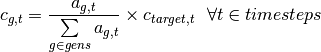where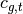is the curtailed power of generator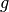in timestep,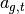is the weather-dependent availability of generatorin timestepand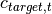is the given curtailment target (power) for timestepto be allocated to the generators.

### ‘voltage-based’¶

The ‘voltage-based’ curtailment is implemented in voltage_based(). The curtailment that has to be met in each time step is allocated to all generators depending on the exceedance of the allowed voltage deviation at the nodes of the generators. The higher the exceedance, the higher the curtailment.

The optional parameter voltage_threshold specifies the threshold for the exceedance of the allowed voltage deviation above which a generator is curtailed. By default it is set to zero, meaning that all generators at nodes with voltage deviations that exceed the allowed voltage deviation are curtailed. Generators at nodes where the allowed voltage deviation is not exceeded are not curtailed. In the case that the required curtailment exceeds the weather-dependent availability of all generators with voltage deviations above the specified threshold, the voltage threshold is lowered in steps of 0.01 p.u. until the curtailment target can be met.

Above the threshold, the curtailment is proportional to the exceedance of the allowed voltage deviation.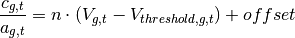whereis the curtailed power of generatorin timestep,is the weather-dependent availability of generatorin timestep,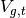is the voltage at generatorin timestepand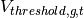is the voltage threshold for generatorin timestep.is calculated as follows: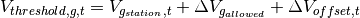where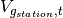is the voltage at the station’s secondary side,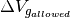is the allowed voltage deviation in the reverse power flow and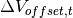is the exceedance of the allowed voltage deviation above which generators are curtailed.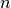and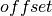in the equation above are slope and y-intercept of a linear relation between the curtailment and the exceedance of the allowed voltage deviation. They are calculated by solving the following linear problem that penalizes the offset using the python package pyomo: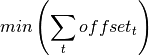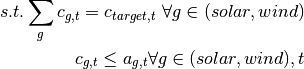whereis the given curtailment target (power) for timestepto be allocated to the generators.

## Storage integration¶

Besides the possibility to connect a storage with a given operation to any node in the grid, eDisGo provides a methodology that takes a given storage capacity and allocates it to multiple smaller storages such that it reduces line overloading and voltage deviations. The methodology is implemented in one_storage_per_feeder(). As the above described curtailment allocation methodologies it is intended to be used in combination with eTraGo where storage capacity and operation is optimized.

For each feeder with load or voltage issues it is checked if integrating a storage will reduce peaks in the feeder, starting with the feeder with the highest theoretical grid expansion costs. A heuristic approach is used to estimate storage sizing and siting while storage operation is carried over from the given storage operation.

A more thorough documentation will follow soon.